Author: Oscar Cronquist Article last updated on May 05, 2022This article demonstrates the XMATCH function introduced in Excel 365. The XMATCH function is an enhanced version of the MATCH function, it also has better default settings not prone to errors.

## 1. How to use the XMATCH function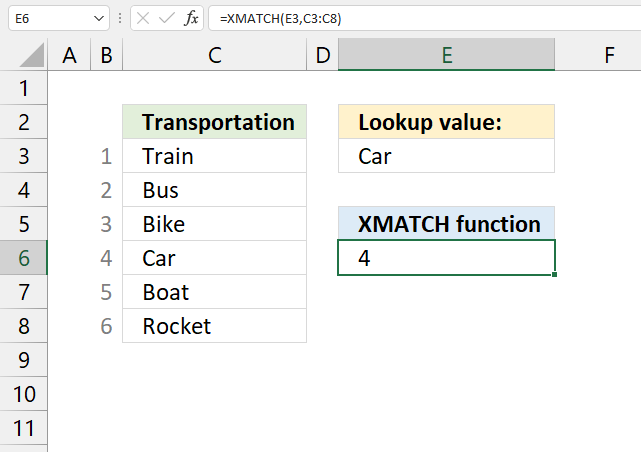The XMATCH function returns the lookup values relative position in a cell range or array. It matches only the first found instance from top to bottom.

Formula in cell D3:

=XMATCH(E3,C3:C8)

The XMATCH function in cell E6 uses the value in cell E3 as a lookup value, the lookup array is cell range C3:C8. The result is 4, the lookup value is the fourth value in cell range C3:C8.

## 2. Excel Function Syntax

XMATCH(lookup_value, lookup_array, [match_mode], [search_mode])

## 3. Arguments

 lookup_value Required. A cell reference or a constant to a lookup value. lookup_array Required. A cell reference or an array to search. [match_mode Optional. Match setting: 0 - Exact match (default value). The XMATCH function returns a #N/A error if an exact match is not found. -1 - Exact match or next smallest item. 1 - Exact match or next largest item 2 - Characters * ? and ~ can be used to perform a wildcard match. [search_mode] Optional. Search setting: 1 - Search first-to-last (default), the XLOOKUP function searches from top to bottom or left to right. -1 - Search last-to-first (reverse search), , the XLOOKUP function searches from bottom to top or right to left. 2 - Binary search, lookup_array must be sorted in ascending order. -2 - Binary search, lookup_array must be sorted in descending order.

Make sure you sort the lookup_array accordingly if you perform a binary search.

## 6. How to return the relative positions of all matching lookup values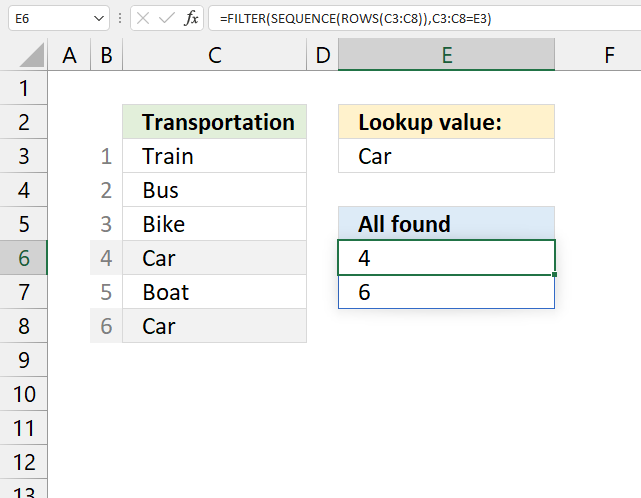This is an Excel 365 dynamic array formula, it contains the FILTER and SEQUENCE functions that exist only in Excel 365.

The image above demonstrates a formula that returns the relative positions of all found instances. For example, lookup value specified in cell E3 is found twice in cell range C3:C8.

The corresponding relative position is 4 and 6, see gray numbers adjacent to cell range C3:C8.

Formula in cell E6:

=FILTER(SEQUENCE(ROWS(C3:C8)),C3:C8=E3)

### 6.1 Explaining formula in cell E6

#### Step 1 - Count values in cell range

The ROWS function counts the number of rows in a cell range or array.

ROWS(C3:C8)

returns 6. There are six rows in cell range C3:C8.

#### Step 2 - Create a sequence

The SEQUENCE function returns an array containing sequence from 1 to n.

SEQUENCE(ROWS(C3:C8))

becomes

SEQUENCE(6)

and returns {1; 2; 3; 4; 5; 6}.

#### Step 3 - Logical test

This step creates a logical expression that will filter corresponding numbers in the next step (step 4).

The equal sign is a logical operator and the output is a boolean value TRUE or FALSE. It compares all values in cell range C3:C8 to value in cell E3.

C3:C8=E3

becomes

{"Train"; "Bus"; "Bike"; "Car"; "Boat"; "Car"}="Car"

and returns

{FALSE; FALSE; FALSE; TRUE; FALSE; TRUE}.

#### Step 4 - Filter numbers based on the lookup value

The FILTER function filters values based on a logical expression.

FILTER(SEQUENCE(ROWS(C3:C8)),C3:C8=E3)

becomes

FILTER({1; 2; 3; 4; 5; 6},{FALSE; FALSE; FALSE; TRUE; FALSE; TRUE})

and returns {4; 6}.

## 7. How to calculate the relative position of column and row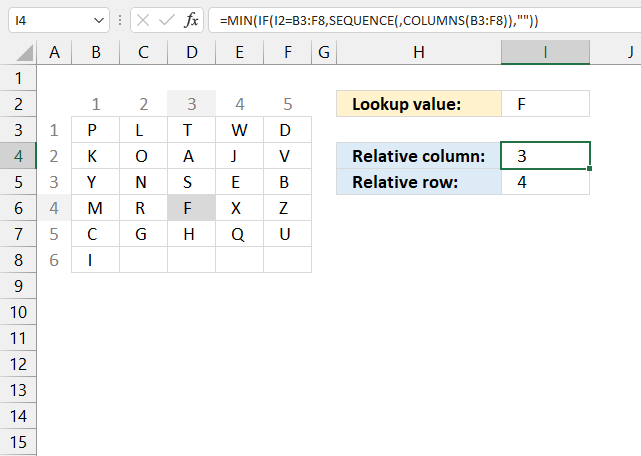This formula calculates the relative position of a matching lookup value in a multi-column cell range.

The image above demonstrates a formula in cell I4 that calculates the relative column number based on the specified lookup value in cell I2.

There is also a second formula in cell I5 that calculates the relative row number.

Formula in cell I4:

=MIN(IF(I2=B3:F8, SEQUENCE(, COLUMNS(B3:F8)), ""))

Formula in cell I5:

=MIN(IF(I2=B3:F8, SEQUENCE(ROWS(B3:F8)), ""))

## 8. Search last to first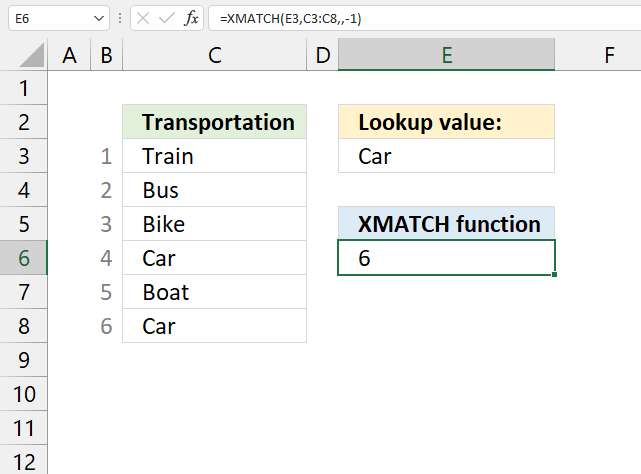This formula demonstrates how to perform a lookup last to first using the XMATCH function.

Formula in cell E6:

=XMATCH(E3, C3:C8, , -1)

The last argument in the XMATCH function lets you specify search mode. -1 tells the function to perform a last to first search.

XMATCH(lookup_value, lookup_array, [match_mode], [search_mode])

## 9. Search right to left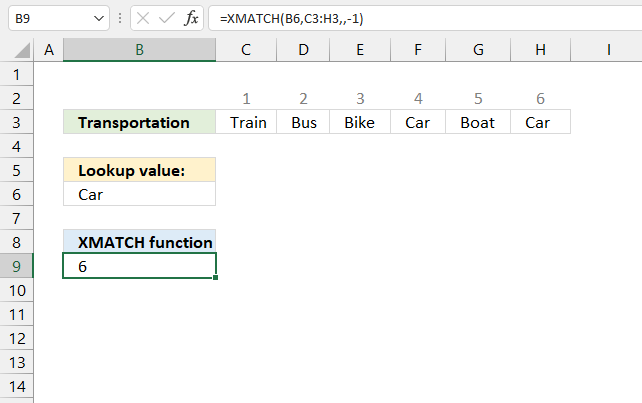This formula demonstrates how to perform a lookup right to left using the XMATCH function.

Formula in cell B9:

=XMATCH(B6, C3:H3, , -1)

The last argument in the XMATCH function lets you specify search mode. -1 tells the function to perform a last to first search, or right to left.

XMATCH(lookup_value, lookup_array, [match_mode], [search_mode])